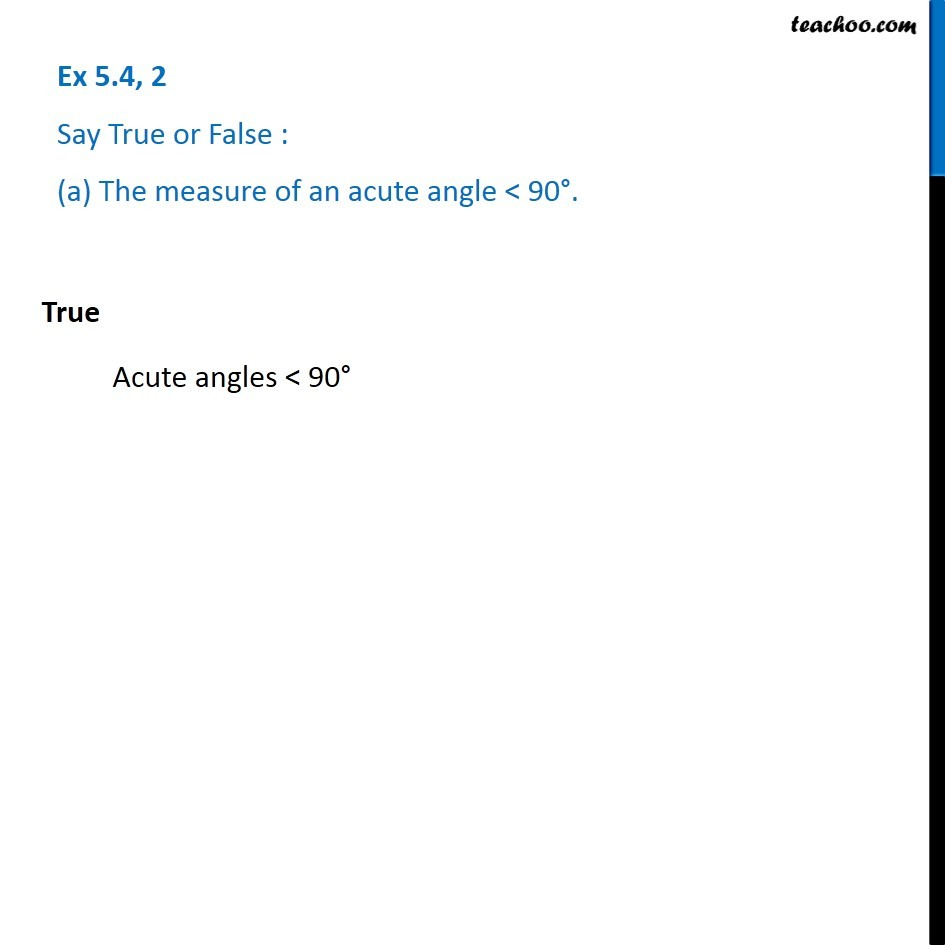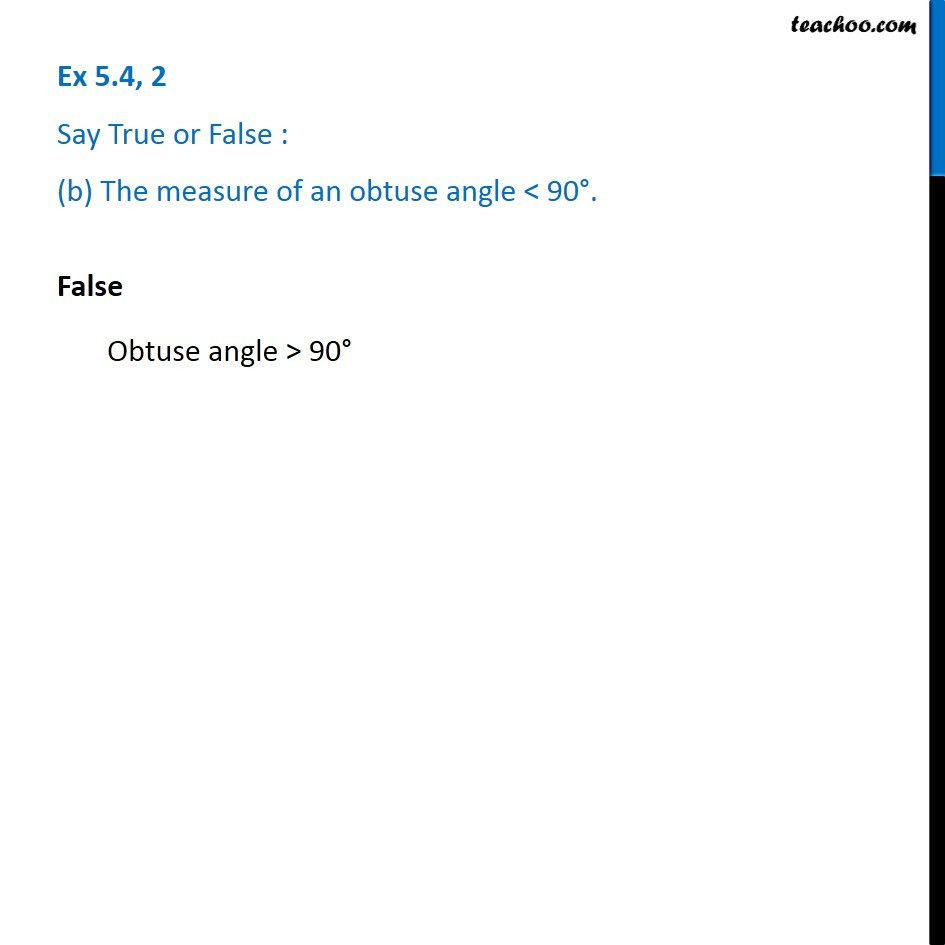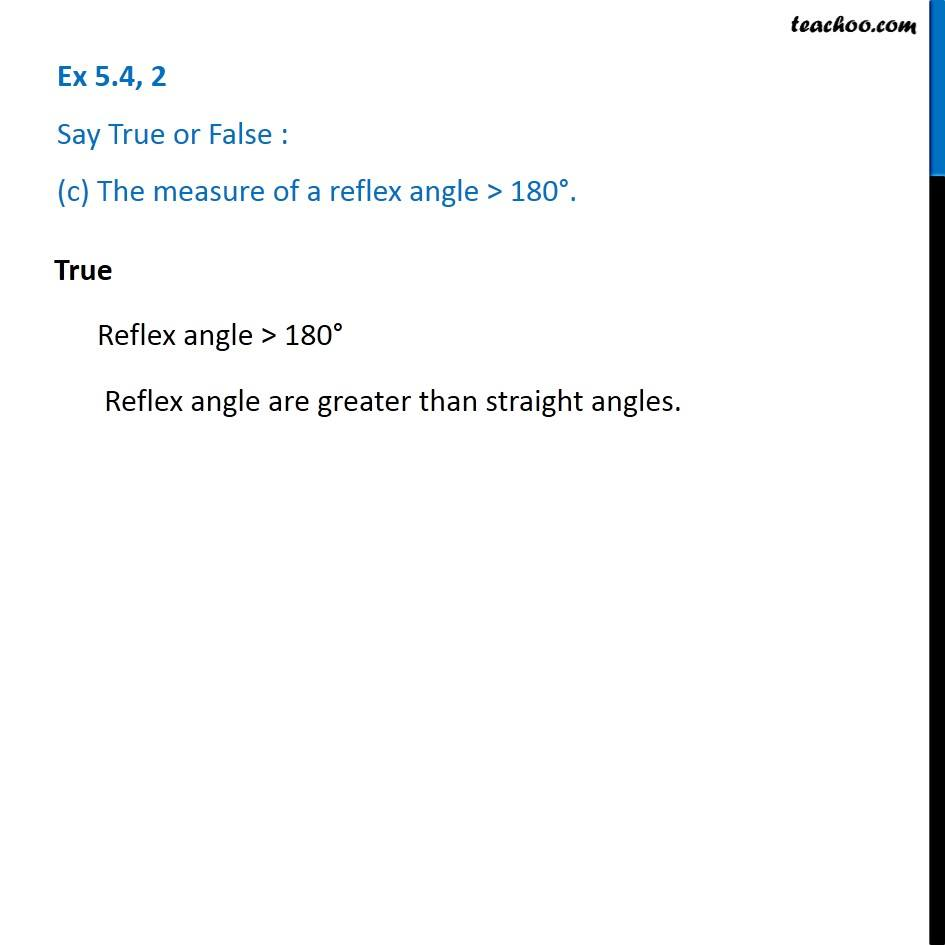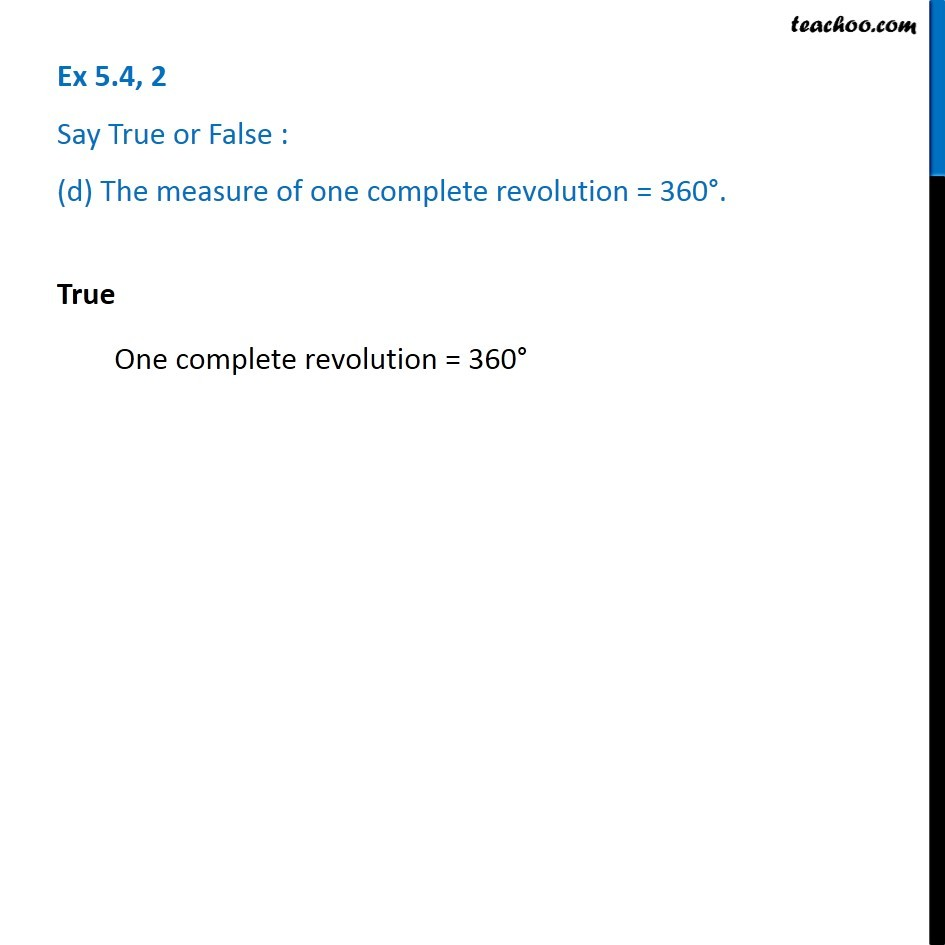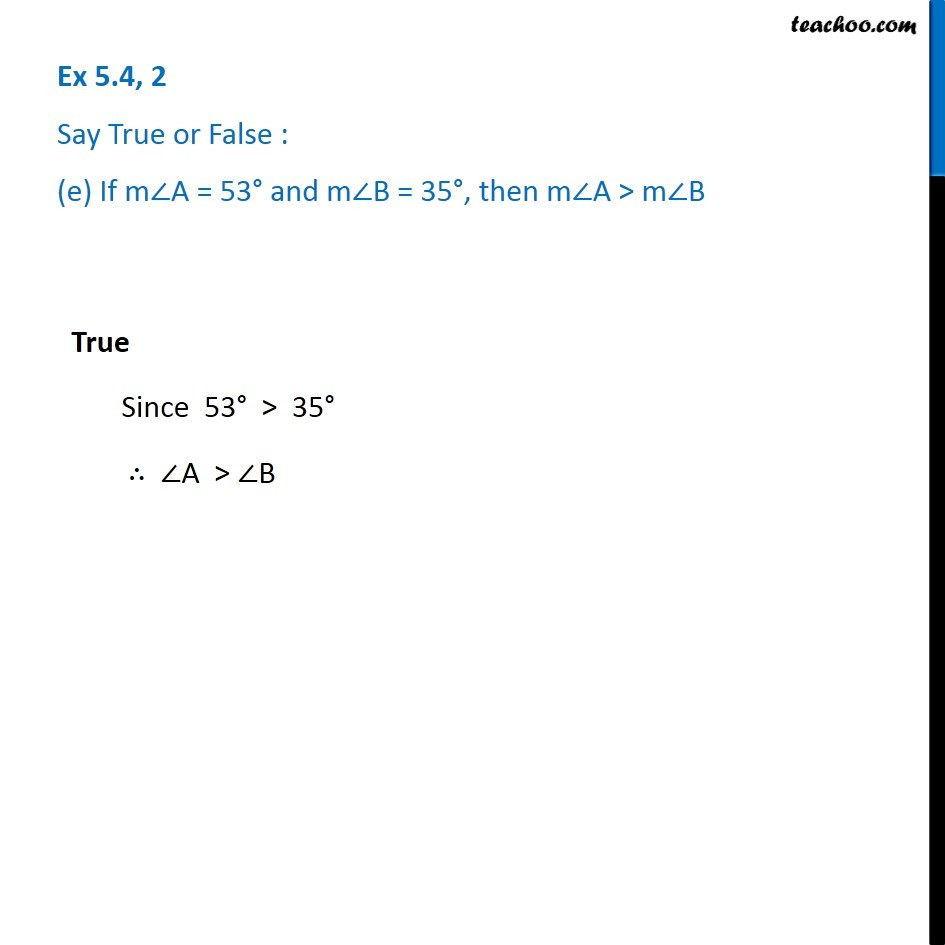1. Chapter 5 Class 6 Understanding Elementary Shapes
2. Concept wise
3. Measuring angles

Transcript

Ex 5.4, 2 Say True or False : (a) The measure of an acute angle < 90°. True Acute angles < 90° Ex 5.4, 2 Say True or False : (b) The measure of an obtuse angle < 90°. False Obtuse angle > 90° Ex 5.4, 2 Say True or False : (c) The measure of a reflex angle > 180°. True Reflex angle > 180° Reflex angle are greater than straight angles. Ex 5.4, 2 Say True or False : (d) The measure of one complete revolution = 360°. True One complete revolution = 360° Ex 5.4, 2 Say True or False : (e) If m∠A = 53° and m∠B = 35°, then m∠A > m∠B True Since 53° > 35° ∴ ∠A > ∠B